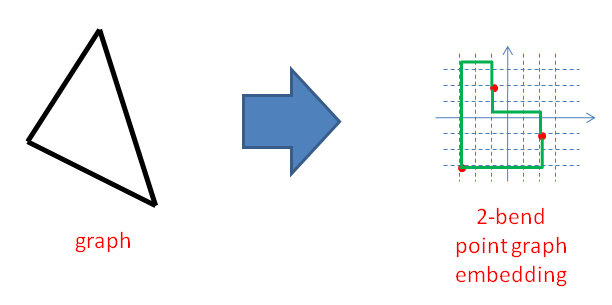## December 2010 riddle

This month's riddle is due to math developed by David Wood. The suggestion that it makes a nice riddle came from Graham Farr. More explicit credits will be given as part of the solution.

Let us define a "point graph embedding" as a mapping of a graph (a set of vertices and connecting edges) into Euclidean Rn space, in such a way that every vertex of the graph is mapped onto a unique point in the space, and every edge is mapped into a path that connects two vertices. Paths are not allowed to overlap or to hit vertex-points, except at their end-points.

A "2-bend point graph embedding" is a special case of such an embedding, where all edges are mapped onto paths that are composed of at most three straight line segments, and each line segment is axis parallel. (It is called a 2-bend point graph embedding because each connecting path bends in at most two points.)

The image below shows an example of such an embedding in two dimensions.This month you are asked to find a 2-bend point graph embedding for the largest cliques that are embeddable in this way in Euclidean space of dimensions 2 through 5. For each of these four spaces (R2, R3, R4 and R5) you are to find the largest embeddable clique and find a point graph embedding for it.

Solutions this month will be checked by a computer, so I ask for a specific format in which to send your answers. The format is as follows:

The embeddings will be provided as file-attachments to your e-mailed answer, one file per embedding. The files will be named "dim2.txt" through "dim5.txt" to denote the solution to 2-dimensional space through 5-dimensional space, respectively. The files will be ASCII text files. Each line will denote a single edge. Each edge will be denoted by a sequence of space-separated point-locations. The path of the edge will be the path composed by joining every two adjacent point locations by a straight line. The first and last point-locations on each line will be the vertices that are the endpoints of the edge. The middle locations, if any, will denote the bends. Be sure not to have more than two bends on a single edge.

Point locations will be denoted as a sequence of n comma-separated Cartesian coordinates (where the space is Rn). No white space is allowed within a point location. Coordinates may be integral or floating point (denoted in ASCII text).

In the body of your answer e-mail, please give a two-sentence proof that larger cliques cannot be embedded in the given dimensions.

To appear on the solvers' list, solve at least 2 of the 4 cases. Different solver lists will be kept for those who sent correct solutions to 2, 3 and 4 of the cases.

### List of solvers:

#### Four cases:

Daniel Bitin (12 December 23:49)
Victor Chang (14 December 13:30)

#### Two cases:

Elegant and original solutions can be submitted to the puzzlemaster at riddlesbrand.scso.com. Names of solvers will be posted on this page. Notify if you don't want your name to be mentioned.

The solution will be published at the end of the month.

Enjoy!